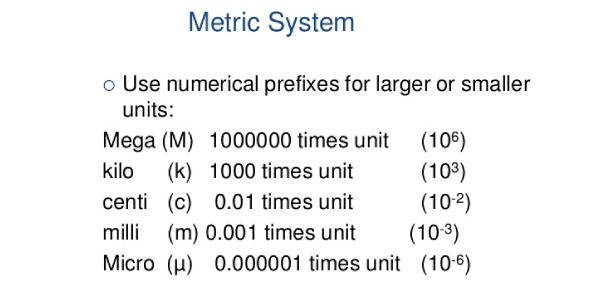# The Metric System Exam! Quiz

47 Questions | Attempts: 530
ShareSettingsAre you well educated regarding the metric system? Do you think you can pass this quiz? Decimal based means that all of the units are based on powers of 10. There are the base units and then a system of prefixes, which may be used to change the base unit by factors of 10. Take this quiz and see if your knowledge of the metric system measures up.

• 1.
5mL = _________L
• 2.
2000mL = ____________L
• 3.
6700uL = _________mL
• 4.
500kL = ____________hL
• 5.
2000mL= ________________uL
• 6.
500km = ____________hm
• 7.
25600mL= ________________L
• 8.
600uL = ______________mL
• 9.
900uL = ______________mL
• 10.
8000uL = ______________mL
• 11.
10mL = ___________L
• 12.
20kL = 200___
• 13.
________kL = 3400L
• 14.
1uL = ________mL
• 15.
67DecaLiter = ____________deciliter
• 16.
0.01hL = ___________cL
• 17.
617kL = 61.7hL
• A.

True

• B.

False

• 18.
0.34kL = 34Decaliter
• A.

True

• B.

False

• 19.
1,500cg  = _____________150kg
• A.

True

• B.

False

• 20.
2.6km = 0.26hm
• A.

True

• B.

False

• 21.
11,000mL = 11uL
• A.

True

• B.

False

• 22.
15cm = 1.5mm
• A.

True

• B.

False

• 23.
18kg = ______________ug
• 24.
18kg = ______________mg
• 25.
18kg = ______________cg

## Related TopicsBack to top
×

Wait!
Here's an interesting quiz for you.